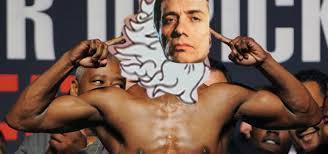Tags
Question
The [...] is the amount by which a change in the monetary base is multiplied to calculate the final change in the money supply.money multiplier

Tags
Question
The [...] is the amount by which a change in the monetary base is multiplied to calculate the final change in the money supply.
?

Tags
Question
The [...] is the amount by which a change in the monetary base is multiplied to calculate the final change in the money supply.money multiplier
If you want to change selection, open original toplevel document below and click on "Move attachment"

#### Parent (intermediate) annotation

Open it
The money multiplier is the amount by which a change in the monetary base is multiplied to calculate the final change in the money supply. Money Multiplier = 1/b, where b is the required reserve ratio. In

#### Original toplevel document

Subject 1. What is Money?
liquid of all assets, due to its function as the medium of exchange. However, many methods of holding money do not yield an interest return and the purchase power of money will decline during a time of inflation. <span>The Money Creation Process Reserves are the cash in a bank's vault and deposits at Federal Reserve Banks. Under the fractional reserve banking system, a bank is obligated to hold a minimum amount of reserves to back up its deposits. Reserves held for that purpose, which are expressed as a percentage of a bank's demand deposits, are called required reserves. Therefore, the required reserve ratio is the percentage of a bank's deposits that are required to be held as reserves. Banks create deposits when they make loans; the new deposits created are new money. Example Suppose the required reserve ratio in the U.S. is 20%, and then suppose that you deposit $1,000 cash with Citibank. Citibank keeps$200 of the $1,000 in reserves. The remaining$800 of excess reserves can be loaned out to, say, John. After the loan is made, the money supply increases by $800 (your$1,000 + John's $800). After getting the loan, John deposits the$800 with Bank of America (BOA). BOA keeps $160 of the$800 in reserves and can now loan out $640 to another person. Thus, BOA creates$640 of money supply. The process goes on and on. With each deposit and loan, more money is created. However, the money creation process does not create an infinite amount of money. The money multiplier is the amount by which a change in the monetary base is multiplied to calculate the final change in the money supply. Money Multiplier = 1/b, where b is the required reserve ratio. In our example, b is 0.2, so money multiplier = 1/0.2 = 5. Definitions of Money There are different definitions of money. The two most widely used measures of money in the U.S. are: The M1 Money Su

#### Summary

status measured difficulty not learned 37% [default] 0

No repetitions